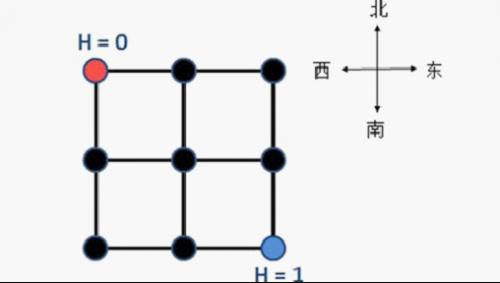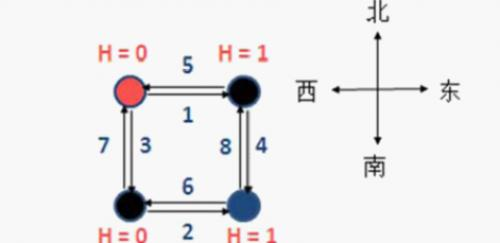# BZOJ2007: [Noi2010]海拔

BZOJ2007: [Noi2010]海拔

## Description

YT市是一个规划良好的城市，城市被东西向和南北向的主干道划分为n×n个区域。## Input

n(n + 1)个数表示所有从西到东方向的人流量，然后n(n + 1)个数表示所有从北到南方向的人流量，n(n + 1)个数表示所有从东到西方向的人流量，最后是n(n + 1)个数表示所有从南到北方向的人流量。

1
1
2
3
4
5
6
7
8

## Sample Output

3

【样例说明】## 题解Here!

$3min$后——

$5min$后——

#include<iostream>
#include<algorithm>
#include<cstdio>
#include<queue>
#define MAXN 260010
#define MAX (1LL<<62)
using namespace std;
int n,s,t,c=1;
long long path[MAXN];
bool vis[MAXN];
struct Edge{
int next,to;
long long w;
}a[MAXN<<3];
int date=0;char c=0;
while(c<'0'||c>'9')c=getchar();
while(c>='0'&&c<='9'){date=date*10+c-'0';c=getchar();}
return date;
}
inline int get_id(int x,int y){return (x-1)*n+y;}
inline int relax(int u,int v,int w){
if(path[v]>path[u]+w){
path[v]=path[u]+w;
return 1;
}
return 0;
}
inline void add(int u,int v,int w){
}
void spfa(){
int u,v;
queue<int> q;
for(int i=1;i<=t;i++){path[i]=MAX;vis[i]=false;}
path[s]=0;
vis[s]=true;
q.push(s);
while(!q.empty()){
u=q.front();
q.pop();
vis[u]=false;
v=a[i].to;
if(relax(u,v,a[i].w)&&!vis[v]){
vis[v]=true;
q.push(v);
}
}
}
printf("%lld\n",path[t]);
}
void init(){
int u,v,w;
s=n*n+1;t=n*n+2;
for(int i=1;i<=n+1;i++)
for(int j=1;j<=n;j++){
u=get_id(i,j);
}
for(int i=1;i<=n;i++)
for(int j=0;j<=n;j++){
u=get_id(i,j);
}
for(int i=1;i<=n+1;i++)
for(int j=1;j<=n;j++){
u=get_id(i,j);
}
for(int i=1;i<=n;i++)
for(int j=0;j<=n;j++){
u=get_id(i,j);